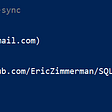# [Cryptohack] Triple DES solution

For this challenge we are provided with the source code for the API we can use:

We have two options:

1. Encrypt arbitrary text with arbitrary key
2. Encrypt flag with arbitrary key

## 3DES introduction

Note, that in this challenge (unlike other challenges in “Block Ciphers” section) we have to work with 3DES. So let’s first see how 3DES works. 3DES is an extension of the old DES algorithm. If you do not know what DES is, you should read about it, it has pretty fascinating history. But for the purpose of this task it is enough to know that DES has several weaknesses. Major DES disadvantage is the key length — 64 bits (actually only 56 bits are used, but not important). DES was actually broken in late 1990s by simple brute force on a special machine within a day or two. This led to the development of a new encryption standard in early 2000s — AES. But there were also some attempts to increase DES security by extending DES. This allowed using earlier developed software and hardware with DES support and prolong life of DES. One of such attempts was 3DES.

The idea behind 3DES is fairly simple: if DES has small key, let’s use 3 different keys and encrypt 3 times. So instead of this

We now have this

Actually second encryption block in 3DES is changed to decryption block

This doesn’t really affect anything, but it is pretty convenient if we need simple DES (we can just set `k1 == k2` and first two blocks will eliminate each other, so effectively text will be encrypted single time with `k3`).

Using 3 different keys increases encryption security only twice (because of the meet in the middle attack), so instead of 56 bit security we get 112 bit, which is actually not bad even for today (but don’t use 3DES, there are other reasons not to do that).

## Challenge solution

Now as we know how 3DES works we can get back to the challenge. Recall that we can encrypt either arbitrary text with arbitrary key, or ask server to encrypt flag for us with arbitrary key. The solution actually might look pretty easy. If we control the key, we can ask server to encrypt the flag with any key, and then just decrypt it by ourselves with the same key. But there is a small problem. Server also uses key whitening technique to increase security.

Key whitening is another approach that was developed in pursue to increase DES security, leading to creation of DES-X. Key whitening is an extremely simple procedure

Although, in this challenge `k1 == k3 == iv`

So now we cannot just decrypt flag with our known key, because the plaintext passed to 3DES is first XORed with `iv`, and later the ciphertext is XORed with `iv`, and we do not know the `iv`. So we have to think of a different approach.

Now it is time to remember, that DES has another weakness — weak keys. Weak keys are the keys that make encryption and decryption operations to be equivalent, i.e. if we twice encrypt text with the weak key, we get the plain text again.

Let’s first illustrate the whole encryption that server performs

`k1, k2, k3` are provided by us. Our attack is the following. Let’s take 3 different weak keys and pass it to flag encryption function, we will get encrypted flag. Now we swap `k3` and `k1` and pass encrypted flag and new keys `k3, k2, k1` to arbitrary text encryption function. Server will encrypt the encrypted flag and we will get the decrypted flag. Let’s break it down by step by step.

1. We ask server to encrypt flag with weak keys `k1||k2||k3`, where `||` means concatenation.
2. Server XORes flag with `iv` -> `p' = flag ^ iv`.
3. `p'` is encrypted with `k1` -> `e1 = E(p', k1)`.
4. `e1` is decrypted with `k2` -> `e2 = D(e1, k2)`.
5. `e2` is encrypted with `k3` -> `e3 = E(e2, k3)`.
6. `e3` is XORed with `iv` -> `e = e3 ^ iv`.
7. Now we take swap `k1` and `k3`, and ask server to encrypt `e` with `k3||k2||k1`.
8. `e` is XORed with `iv` -> `e3 = e ^ iv` (because `e = e3 ^ iv`, `e3 = e ^ iv = e3 ^ iv ^ iv = e3 ^ 0 = e3`).
9. `e3` is encrypted with `k3`, but `k3` is a weak key, so effectively encryption is the same as decryption with `k3` -> `e2 = E(e3, k3) = D(e3, k3)`.
10. `e2` is decrypted with `k2` -> `e1 = D(e2, k2) = E(e2, k2)`.
11. `e1` is encrypted with `k1` -> `p' = E(e1, k1) = D(e1, k1)`.
12. Finally, `p'` is XORed with `iv` which yields the flag `flag = p' ^ iv = flag ^ iv ^ iv = flag ^ 0 = flag`.

You can find weak keys for DES here https://en.wikipedia.org/wiki/Weak_key

And here is the implementation:

--

--

--

## More from 0awawa0

awawa.fun

Love podcasts or audiobooks? Learn on the go with our new app.

## HOW DOES DARK WEB HELP CYBER SECURITY EXPERTS IMPROVE BUSINESS SECURITY?## DAFI Protocol and SolanaPrime Partner To Help Transform The Web3 Industry## An Introduction To The Implications Of Iran’s Cyber Capabilities For The US## Stop Calling People — Do This Instead## Browsing History in Bitdefender DBs## How accurate can IP Geolocation get?## ElasticDAO Smart Contract and Security Auditsawawa.fun

## Nakji Network’s 200K Bug Bounty Program## Magic HTB## TryHackMe Writeup : Solar, exploiting Log4J🥷🏻🥷🏻## “Tricking” our developers into liking application security